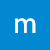# Physics equations and the things behind them

Mind Map by , created 9 months ago

## Mind Map on Physics equations and the things behind them, created by Matthew Roderick on 02/06/2019.300Created by Matthew Roderick 9 months ago
Physics Review!
Physics: Energy resources and energy transfer
P1 - The Earth in the Universe
Higher Level Economics
Epithelial tissue
Forces and motion
Physics 1A - Energy
Physics P1
OCR Physics P2 revision cards
Physics equations and the things behind them
1 density(kg/m³)=mass(kg)/volume (m³)
1.1 it does not vary with shape or size
1.2 what it is made up of
1.3 determines if it floats or sinks
1.4 experiments
1.4.1 you can measure it in solids or liquids
1.4.2 you can use a measuring cylinder to measure liquid density
1.4.2.1 1ml = 1cm³ then use the mass to calculate it
1.4.3 solid cuboid
1.4.3.1 measure length width height and multiply together
1.4.4 solid cylinder
1.4.4.1 diameter of circles at the bass halve to get r
1.4.4.1.1 volume=π X r² X height
1.4.5 eureka can
1.4.5.1 fill up to just the spout
1.4.5.1.1 the object you put in the can will displace the exact volume of itself so use 1ml = 1cm³
1.4.5.1.1.1 repeat 3 times and figure out a mean before calculating
1.4.5.2 let it drain before using the measuring cylinder
1.4.5.3 thin string should be used to get the most accurate reading
2 distance travled(m) = speed(m/s) X time(s)
3 acceleration(m/s²) = change in velocity(m/s) / time(s)
4 kinetic energy(j) = 0.5 X mass(kg) X (speed(m/s)²)
5 moles = actual mass/ molar mass